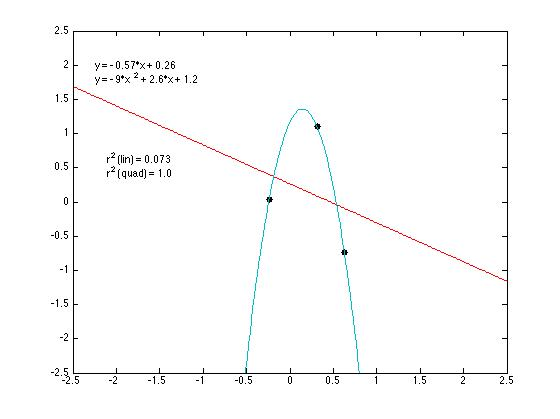## darb.ketyov.com

Caveat lector: This blog is where I try out new ideas. I will often be wrong, but that's the point.

## 11.12.13

### An intuitive explanation of over-fitting

A question over on Quora piqued my interest: What is an intuitive explanation of over-fitting? I use this blog and public writing to try and explain neuroscience topics, but I don't think I've ever taken a whack at explaining statistics. Which now strikes me as strange considering how much of my research is computational and methods-focused. So I gave it a go. Let me know if this makes sense.

Imagine you've got two normally distributed random variables, x and y. Here is their relationship:When you know all of the data, it should be clear that knowledge of the value of x provides little information about y. That is, there is no clear predictive relationship. Certainly not a linear one.

We can test this by trying to fit a linear model (y = ax + b) to the data (the red line). This procedure shows us that the variance in x accounts for less than 1% of the variance in y.

But let's imagine you can't collect all of the data. Instead your sample includes only two of those data points. Again you try and fit a linear model and...Holy crap! Your linear model (y = ax + b) explains 100% of the variance! Now you might say, "my linear model is perfect and completely explains the relationship between x and y". But wait... that fitting estimate doesn't look the same as the linear fit when we've got the full data:In fact, our "perfect" model is pretty terrible!

That is overfitting. When your model has too many parameters relative to the number of data points, you're prone to overestimate the utility of your model.

We can keep going with this:When your sample contains three data points, our linear model (y = ax + b) once again performs poorly (~7% of the variance) but our new model, a quadratic model (y = ax^2 + bx + c) explains 100% of the variance.

Again, sampling one more data point:Well now our linear and quadratic models both perform poorly, but our new new model, a cubic model (y = ax^3 + bx^2 + cx + d), explains 100% of the variance.

And so on.